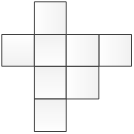### Sample Problem

The figure is made up of 8 identical squares. Its area is 72 cm2. What is its perimeter?Its perimeter is cm.

#### Solution

Area of 8 squares = 72 cm2

Area of 1 square = 72 cm2 ÷ 8

= 9 cm2

Side of 1 square = 3 cm

Number of sides = 16

∴ Perimeter = 16 × 3 cm

= 48 cm

Thus, the perimeter of the figure is 48 cm.